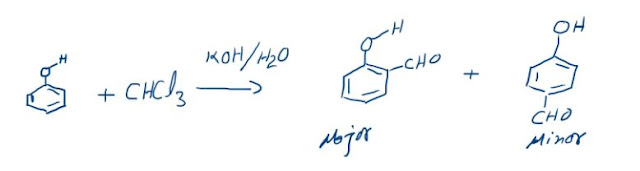## LIQUEFACTION OF GASES

LIQUEFACTION OF GASES:When the molecules of a gas are brought closer to each other due to increase in the attractive forces a stage may be reached when the gas changes its phase to liquid. This phenomenon is called Liquefaction of gases. Obviously, an ideal gas cannot be liquefied as there are no interactions in that case. For the liquefaction of Real gases, we can do either of the following:
1.     Increase the pressure on the gas
2.     Decrease the Temperature of the gas
Both these processes will tend to bring the molecules closer and liquefaction may take place. But it is observed practically that Temperature is the dominant factor for liquefaction and pressure assumes a secondary role. For every real gas a temperature is observed above which it cannot be liquefied even on the application of very high pressures. This characteristic temperature of a real gas above which it cannot be liquefied is called “Critical Temperature” represented by TC. To understand this concept in depth, observe the isotherms drawn in the following figure for a real gas at varying temperatures.

## LIQUEFACTION OF GASES

We observe the following:
1.     At high Temperatures like T4, the isotherms look just like those of ideal gas.
2.     At low temperatures the curve is entirely different. For example, curve abcd at temperature T1. As the pressure increases the volume of the gas decreases from point a to b. Then there is a sudden reduction in volume without much increase in pressure of the gas from b to c. In this portion liquefaction is taking place at constant pressure and due to the difference in the properties of gas and liquid the volume is reducing so much. And after c even on increasing pressure there is no appreciable decrease in volume as now only liquid is present. So, ab represents gaseous phase, bc represents liquid-gas coexistence and cd represents liquid phase.
3.     On carrying out the same process at a higher temperature we observe that the width of the horizontal portion decreases and the pressure at which liquefaction takes place also becomes higher. The horizontal portion decreases because at higher temperatures the properties of liquid approach that of a gas and ΔV = VG – VL reduces.
4.     At temperature TC the horizontal portion reduces to a mere point at which liquefaction takes place. Point Y is called critical point ; at this point the boundary between liquid and gas phase (meniscus) disappears indicating that both the phases have identical characteristics.
5.     Above this temperature there is no liquefaction of gases and gas behaves almost ideally.
At critical point Y the tangent is horizontal , so that , dP/dV at critical point will be zero.Thus , the point Y is also called the point of deflection.

## CRITICAL CONSTANTS

Critical temperature, TC is the maximum temperature at which a gas can be liquefied that is a temperature above which liquid cannot exist.
Critical Pressure, PC is the minimum pressure required for liquefaction to take place at the critical condition.
Critical volume, VC is the volume occupied by one mole of the gas at critical temperature and critical pressure.
These parameters are given by the following expressions:
TC = 8a/27Rb
PC = a/27b2
VC = 3b

Discovery of critical phenomenon by Andrew in 1869 showed that gases can not be liquefied by the application of pressure alone; they must be cooled below their critical temperatures and then subjected to adequate pressure to cause liquefaction.
Principles involved in Liquefaction are :
1. a gas must be at below or belowits critical temperature. lower the temperature below the critical  value , easier would be the liquefaction.
2. The gas is cooled either by doing external work or by expanding against the internal forces of molecular attraction.
Lower temperature for liquefaction of gases can be achieved by the following  techniques :
(a) Cooling by rapid evaporation of a volatileliquid.
(b) Cooling by joule-thomson effect.
(c) Cooling by the adiabatic expansion involving mechanical work

### The Reimer-Tiemann reaction: Reaction and its Mechanism

Reimer-Tiemann Reaction Phenols on reaction with chloroform in the presence of sodium hydroxide (or potassium hydroxide) solution give hydro...